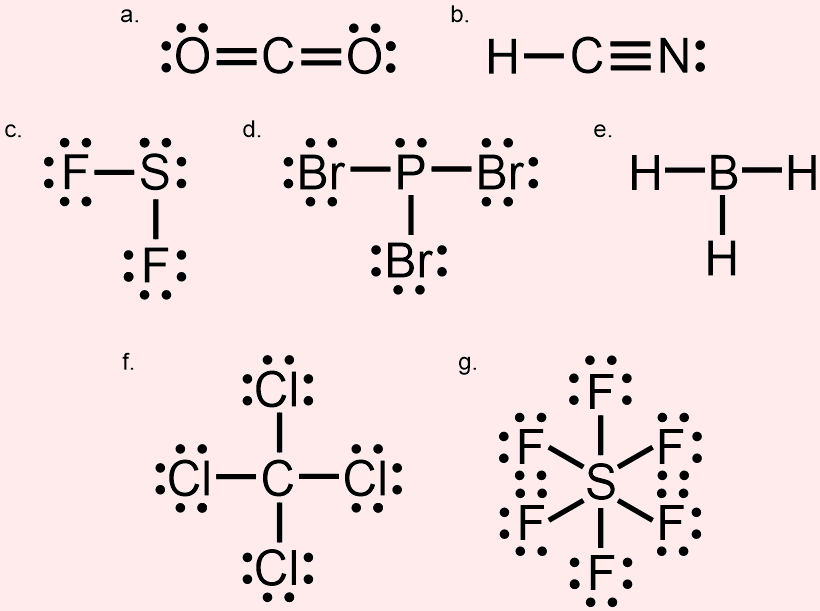# 3.22: Covalent Bonding: Determining the VSEPR Shapes of Molecules

$$\newcommand{\vecs}{\overset { \rightharpoonup} {\mathbf{#1}} }$$ $$\newcommand{\vecd}{\overset{-\!-\!\rightharpoonup}{\vphantom{a}\smash {#1}}}$$$$\newcommand{\id}{\mathrm{id}}$$ $$\newcommand{\Span}{\mathrm{span}}$$ $$\newcommand{\kernel}{\mathrm{null}\,}$$ $$\newcommand{\range}{\mathrm{range}\,}$$ $$\newcommand{\RealPart}{\mathrm{Re}}$$ $$\newcommand{\ImaginaryPart}{\mathrm{Im}}$$ $$\newcommand{\Argument}{\mathrm{Arg}}$$ $$\newcommand{\norm}{\| #1 \|}$$ $$\newcommand{\inner}{\langle #1, #2 \rangle}$$ $$\newcommand{\Span}{\mathrm{span}}$$ $$\newcommand{\id}{\mathrm{id}}$$ $$\newcommand{\Span}{\mathrm{span}}$$ $$\newcommand{\kernel}{\mathrm{null}\,}$$ $$\newcommand{\range}{\mathrm{range}\,}$$ $$\newcommand{\RealPart}{\mathrm{Re}}$$ $$\newcommand{\ImaginaryPart}{\mathrm{Im}}$$ $$\newcommand{\Argument}{\mathrm{Arg}}$$ $$\newcommand{\norm}{\| #1 \|}$$ $$\newcommand{\inner}{\langle #1, #2 \rangle}$$ $$\newcommand{\Span}{\mathrm{span}}$$$$\newcommand{\AA}{\unicode[.8,0]{x212B}}$$

Learning Objective
• Predict the Valence Shell Electron Pair Repulsion, or VSEPR, shape of a covalent molecule, based on the bonds and lone pairs associated with the central atom of a Lewis structure.

The Lewis structures of many covalent molecules have been drawn and discussed in the last several sections of this chapter.  While Lewis structures are relatively simple to construct and accurately reflect the connectivity of atoms within covalent molecules, this drawing convention is otherwise limited in its utility.  In particular, because the world is filled with three-dimensional objects, Lewis structures, which are two-dimensional pictures, by definition, do not correctly depict the spatial orientations of covalent molecules or their constituent atoms.  As a result, an alternative structural notation was developed, in order to properly illustrate the true three-dimensional shapes of covalent molecules.

## Valence Shell Electron Pair Repulsion Notation

Covalent molecules are formed when the unpaired valence shell electrons found within two atoms interact to form shared pairs of electrons.  Because electrons are negatively-charged subatomic particles, the presence of multiple electrons within the bonds and lone pairs of a molecule is moderately destabilizing, as identically-charged particles repel one another when in close proximity.  Consequently, increasing the distance between these bonds and lone pairs will minimize the effects of these repulsive forces and improve the overall stability of the corresponding molecule.

Representing a covalent molecule as a two-dimensional Lewis structure inherently restricts the degree to which electron pairs can be separated.   Valence Shell Electron Pair Repulsion, or VSEPR, notation does not have any spatial restrictions and, therefore, can be utilized to depict molecules using three-dimensional structures.  For this reason, VSEPR pictures more accurately represent the true shapes of covalent molecules, relative to Lewis structures, as three-dimensionally distributing the bonds and lone pairs that are present in a molecule maximizes their physical separation.

In order to represent the three-dimensional directions in which bonds can be oriented within covalent molecules, VSEPR notation uses three distinctive symbols, which are shown below in Figure $$\PageIndex{1}$$.  The left-most symbol in Figure $$\PageIndex{1}$$ is the line that has been used to represent covalent bonds in the previous sections of this chapter.  This symbol, which is known as a stick bond, does not convey any three-dimensional information.  The middle image in Figure $$\PageIndex{1}$$, which is called a wedged bond, indicates that the that is atom located on the wider end of the symbol is oriented toward the viewer, relative to the atom that is found on the pointed end of the bond.  The right-most image in Figure $$\PageIndex{1}$$, which is known as a dashed bond, denotes that the atom that is located on the wider end of the symbol is oriented away from the viewer, relative to the atom that is found on the pointed end of the bond.Figure $$\PageIndex{1}$$: VSEPR bond symbolisms.

The most common three-dimensional shapes of covalent molecules are illustrated below in Figure $$\PageIndex{2}$$.  These pictures, which are labeled with their corresponding VSEPR terms, utilize the bonding symbols in Figure $$\PageIndex{1}$$ to indicate the three-dimensional orientations of each molecule's constituent atoms, which are generically symbolized by the placeholder letters "Q," "A," and "Z."  In each case, "Q" represents the central atom that is present in the molecule, and "A" and "Z" are used to denote unique surrounding atoms.Figure $$\PageIndex{2}$$: Common three-dimensional shapes of covalent molecules and their corresponding VSEPR terms.

The bonds and lone pairs that are oriented around the central atom in a molecule, which is represented by "Q" in Figure $$\PageIndex{2}$$, both experience and generate more significant repulsive forces than those electrons that are located on surrounding atoms.  As a result, the three-dimensional VSEPR shape of a molecule is primarily dependent on the combination of bonds and lone pairs that are associated with its central atom.

For example, consider the orange carbon in the Lewis structure shown below.  This carbon is associated with one single bond and one triple bond.  Therefore, based on the structures shown in Figure $$\PageIndex{2}$$, the VSEPR shape of this molecule at the indicated carbon is linear.Example $$\PageIndex{1}$$

Predict the VSEPR shape of the orange carbon in the Lewis structure shown below.Solution

This carbon is associated with two single bonds and one double bond.  Therefore, based on the structures shown in Figure $$\PageIndex{2}$$, the VSEPR shape of this molecule at the indicated carbon is trigonal planar.

Exercise $$\PageIndex{1}$$

Predict the VSEPR shape of each of the molecules shown below.The central atom in this molecule, a carbon (C), is associated with two double bonds.  Therefore, based on the structures shown in Figure $$\PageIndex{2}$$, the VSEPR shape of this molecule is linear.
The central atom in this molecule, a carbon (C), is associated with one single bond and one triple bond.  Therefore, based on the structures shown in Figure $$\PageIndex{2}$$, the VSEPR shape of this molecule is linear.
The central atom in this molecule, a sulfur (S), is associated with two single bonds and two lone pairs.  Based on the structures shown in Figure $$\PageIndex{2}$$, the VSEPR shape of this molecule is bent.
The central atom in this molecule, a phosphorus (P), is associated with three single bonds and one lone pair.  Based on the structures shown in Figure $$\PageIndex{2}$$, the VSEPR shape of this molecule is trigonal pyramidal.
The central atom in this molecule, a boron (B), is associated with three single bonds.  Based on the structures shown in Figure $$\PageIndex{2}$$, the VSEPR shape of this molecule is trigonal planar.